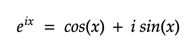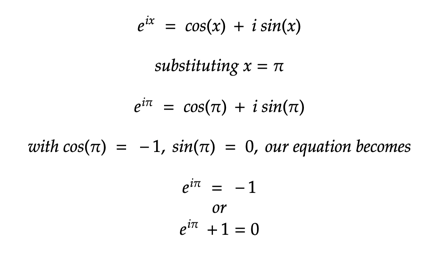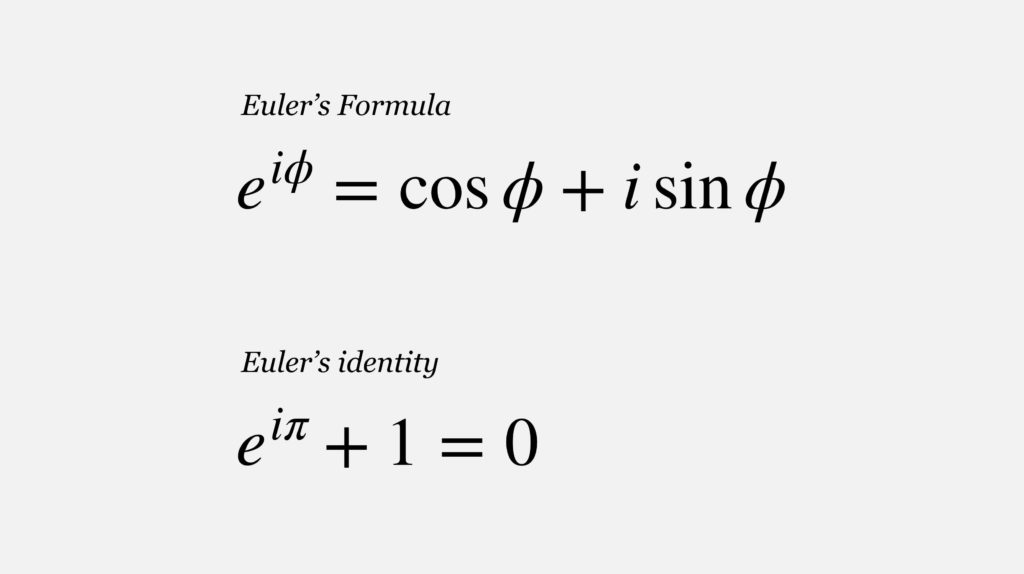# Deriving the famous Euler’s formula through Taylor Series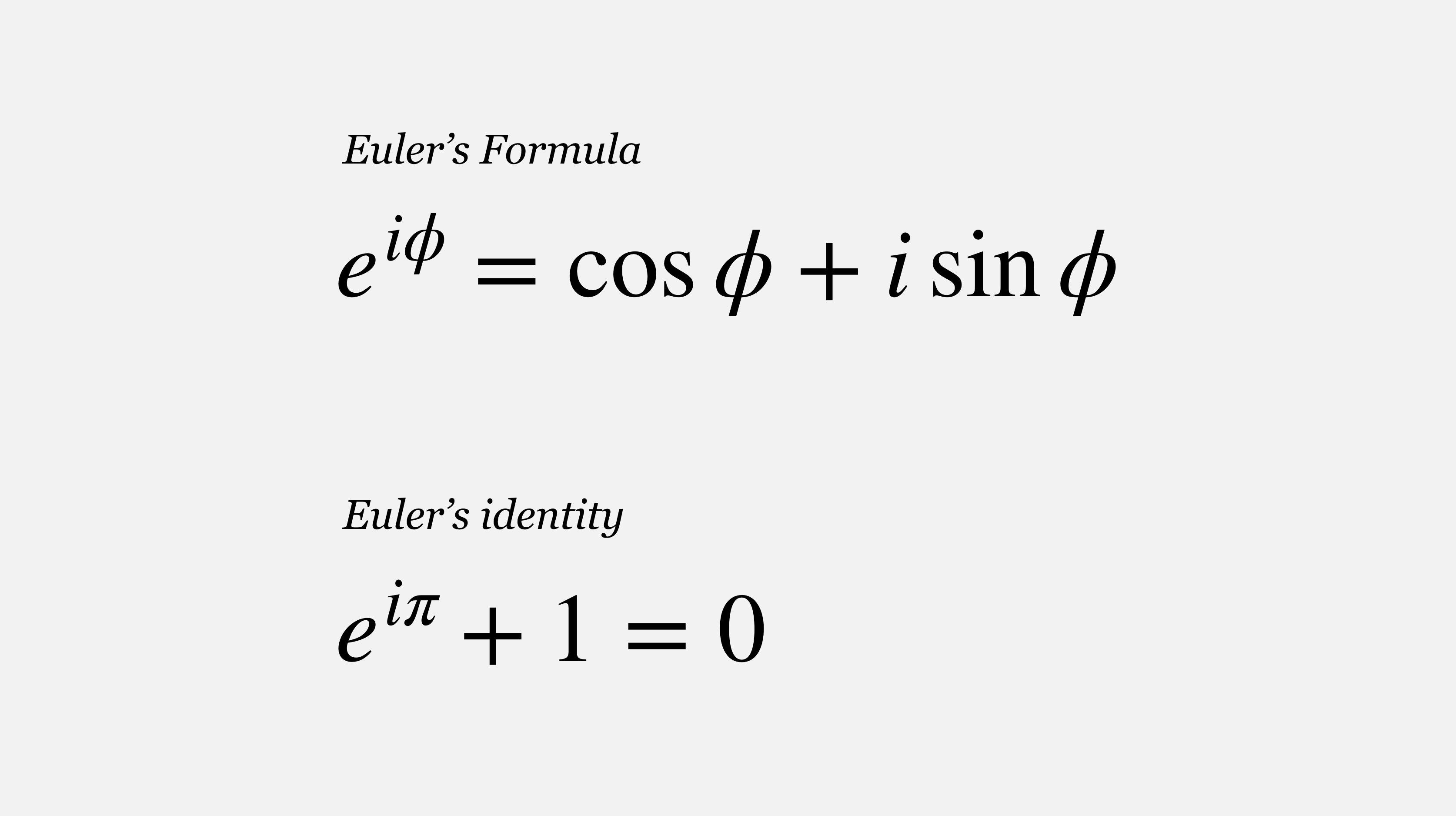Euler’s formula is often coined the most remarkable formula in mathematics. It combines the seemingly unrelated exponential functions, imaginary numbers, and trigonometric functions into a single aesthetically pleasing and beautiful equation. We see five numbers (e, i, π, 0, and 1) that we are familiar with as well as three simple operations (exponentiation, multiplication, and addition) that we use frequently in mathematics. Euler’s Identity takes many concepts that are seemingly unrelated and puts them together in one equation that we can make sense of.

The great physicist Richard Feynman called the Euler formula “our jewel” and “one of the most remarkable, almost astounding, formulas in all of mathematics.”

## Derivation

The Euler’s formula can be easily derived using the Taylor series which was already known when the formula was discovered by Euler. Taylor series is a representation of a function as an infinite sum of terms that are calculated from the values of the function’s derivatives at a single point. The Taylor expansion for some well known functions are: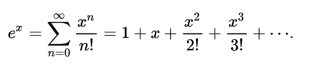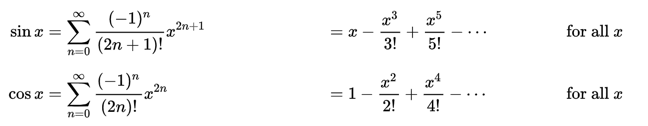source: wikipedia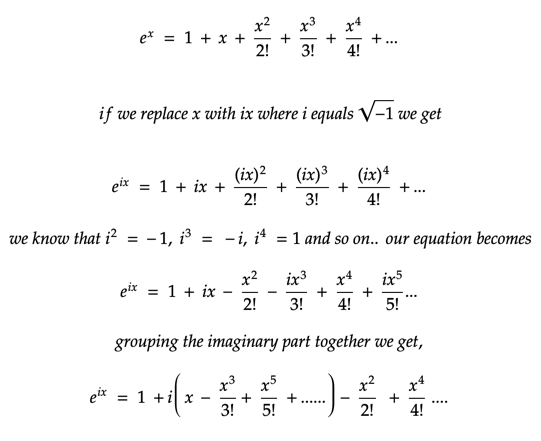If you look at the equations after grouping the imaginary parts of the series, you would see it resembles the Tayler series expansion of Sin(x) and Cos(x). Now our equation can be written in a much shorter form as: